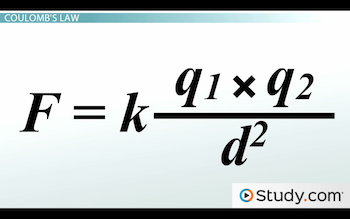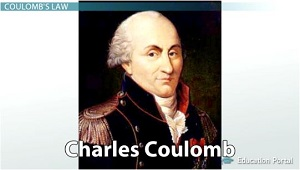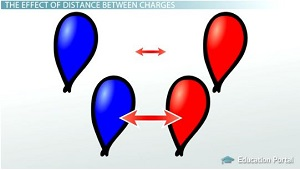# Coulomb's Law: Variables Affecting the Force Between Two Charged Particles

An error occurred trying to load this video.

Try refreshing the page, or contact customer support.

Coming up next: Electric Potential: Charge Collections and Volt Unit

### You're on a roll. Keep up the good work!

Replay
Your next lesson will play in 10 seconds
• 0:05 Coulomb's Law
• 0:59 The Effect of Particle Charge
• 3:24 The Effects of…
• 5:43 The Combined Effects…
• 6:21 Coulomb as the Unit of Charge
• 7:06 Lesson Summary

Want to watch this again later?

Timeline
Autoplay
Autoplay
Speed

#### Recommended Lessons and Courses for You

Lesson Transcript
Instructor: Jim Heald

Jim has taught undergraduate engineering courses and has a master's degree in mechanical engineering.

In the 18th century, Charles Coulomb uncovered the secrets of electrostatic force between charged particles. The results of his experiments led to what is now known as Coulomb's Law, which tells us how force, charge, and distance are all related.

## Coulomb's LawBack in the 18th century, it was well known that an electrically charged particle would exert a force on any other charged particle. The problem was no one knew how strong the force was or what factors affected its strength. That is, until a very bright scientist by the name of Charles Coulomb conducted several experiments that led him to propose what is now known as Coulomb's law.As we'll discuss in this lesson, he found that the force between charged particles was dependent on only two factors: the distance between the particles and the amount of electric charge that they carried. We won't worry about memorizing the equation, but we will focus instead on understanding what the equation tells us about the relationships between force, distance, and charge.

## The Effect of Particle Charge

The force between charged particles is directly related to the amount of charge carried by each particle. Aside from electrons and protons, most charged particles carry a variable amount of charge. Think of rubbing a balloon on your hair. The balloon will pick up negative charges from your hair and begin to act like one big charged particle. The amount of charge on the balloon will depend on how long you rub it on your hair. Now, if you repeat this experiment with a second balloon, the two will try to repel each other, and the strength of that repelling force will depend on how much charge each balloon picked up from your hair.

Going back to Coulomb's equation, we can see that the amounts of charge carried by each balloon are represented by the terms q1 and q2. To allow us to focus on the relationship between the force and the charges, let's strip away the other parts of the equation. What this part of the equation tells us is that the force is directly related to the product of the two charges. In other words, if the product of the charges increases, then the force will also increase. To really understand what this means, let's try some examples.

We'll start out with equal amounts of charge on each balloon, which results in a repelling force between them. If we double the amount of charge on one of the balloons, then the product of the charges will also double, and in turn, the force will double. Next, let's double the amount of charge on the other balloon and see what happens. The product is now four times larger than it was to begin with, which means the force will also be four times larger.

To demonstrate why it's the product of the charges that's important, as opposed to the individual charges, let's go back to the beginning. This time, we'll double the charge on one balloon but cut the charge in half on the other balloon. When we do the math, we can see that the product remains the same, which means that the force will be unchanged. This is why it's never safe to assume that just because the charge on one particle changes, that the force will change as well.

## The Effect of Distance Between Charges

The force between charged particles is very dependent on the distance between them, even more so than on the particle charges we just discussed. In Coulomb's equation, the distance between particles is represented by the term d. To allow us to focus on the relationship between the force and the distance, let's strip away the other parts of our equation. We can see that the distance term is on the bottom of the fraction, which tells us that the force and the distance are inversely related. In other words, if the distance increases, then the force will decrease. Or if the distance decreases, then the force will increase. Force and distance always change opposite of one another.The next thing to notice is that the distance term is squared, which is really just another way to say 'distance multiplied by distance.' Written like this, it becomes clear that the distance is actually represented twice in the equation. This tells us that a change in the distance will have more effect on the force than the same amount of change in the particle charges. To help clarify, let's look at some more examples.

To unlock this lesson you must be a Study.com Member.

### Register to view this lesson

Are you a student or a teacher?

### Unlock Your Education

#### See for yourself why 30 million people use Study.com

##### Become a Study.com member and start learning now.
Back
What teachers are saying about Study.com

### Earning College Credit

Did you know… We have over 160 college courses that prepare you to earn credit by exam that is accepted by over 1,500 colleges and universities. You can test out of the first two years of college and save thousands off your degree. Anyone can earn credit-by-exam regardless of age or education level.